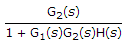# Electronics and Communication Engineering - Automatic Control Systems

### Exercise :: Automatic Control Systems - Section 2

11.

For the system in the given figure, the transfer function C(s)/R(s) is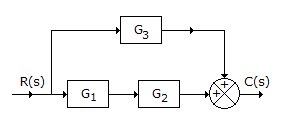A. G1 + G2 + G3 B. G1 G2 + G3 C. G1 G2 G3 D. (G1 + G2) G3

Explanation:

12.

For the system in the given figure, the characteristic equation is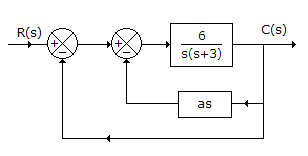A. s2 + s(3 + 6a) + 6 = 0 B. s2 + s(3 + 12a) + 12 = 0 C. s2 + 3s = 0 D. s2 + 6s = 0

Explanation:

Transfer function to inner loop =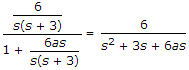.

Then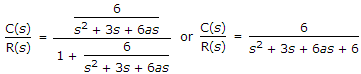.

The characteristic equation is s2 + 3s + 6as + 6 = 0.

13.

In control systems the magnitude of error voltage

 A. is very small B. is very large C. neither small nor large D. may be any value from 0 to 50 V

Explanation:

The error voltage is always very small. A high value of error voltage may cause instability.

14.

The slope of log-magnitude asymptote changes by - 40 dB/ decade at a frequency ω1. This means that

 A. a double pole is present B. a pair of complex conjugate poles is present C. either a double pole or a pair of complex conjugate poles is present D. a pole or zero at origin is present

Explanation:

A double pole or pair of complex poles result in - 40 dB/decade slope in log magnitude graph.

15.

In the given figure the transfer function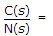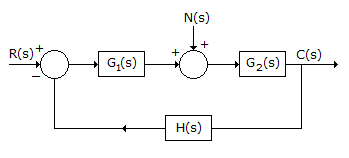A.B.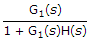C.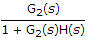D.